﻿ 一种低散射金属支架的运动学计算及仿真 Kinematic Calculation and Simulation of a Low Scattering Metal Pylon

Mechanical Engineering and Technology
Vol.06 No.01(2017), Article ID:20136,10 pages
10.12677/MET.2017.61010

Kinematic Calculation and Simulation of a Low Scattering Metal Pylon

Jiaxing Hou, Wuyi Chen

School of Mechanical Engineering and Automation, Beihang University, Beijing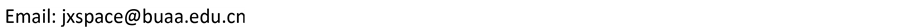Received: Mar. 11th, 2017; accepted: Mar. 28th, 2017; published: Mar. 31st, 2017ABSTRACT

A low scattering metal pylon, mainly used for target supporting in electromagnetic scattering measurement, is important to design and manufacture stealth weapons. The target positioning structure and overall stiffness of the pylon are very important when the RCS measurement is carried out in the compact range. This paper analyzed the structural characteristics of an oval metal pylon and calculated position parameters of the space rotating shaft. We obtained the curve of motion by mechanical analyses under a 200 Kg load and a kinematic simulation using ADAMS, and the curve provides an effective reference for the design and manufacture of driving devices of such metal pylons.

Keywords:Electromagnetic Scattering, Metal Pylon, Kinematics Calculation, Simulation Analysis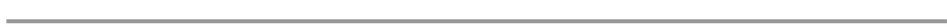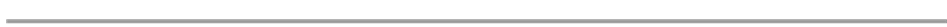1. 引言

2. 低散射金属支架的基本结构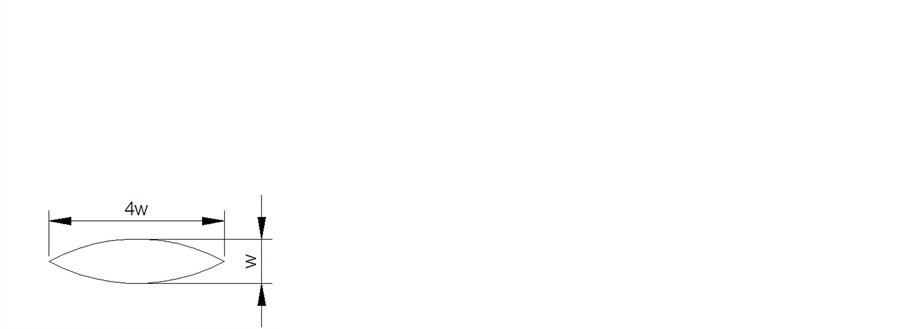(a) 金属支架基本结构 (b) 橄榄形截面形状

Figure 1. The structure of metal pylon

3. 支架空间转轴的计算

3.1. 计算欧拉角、欧拉旋转矩阵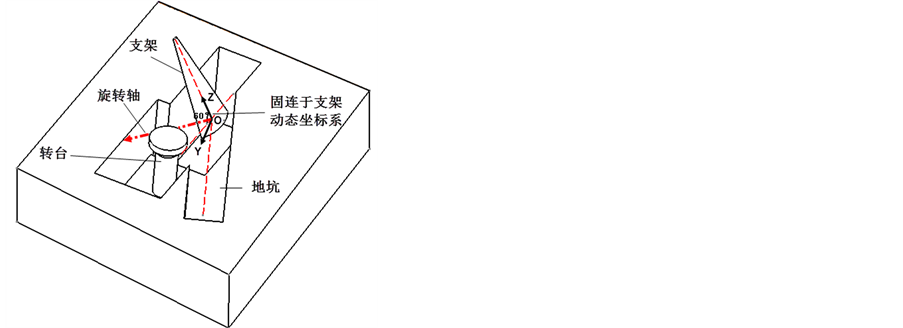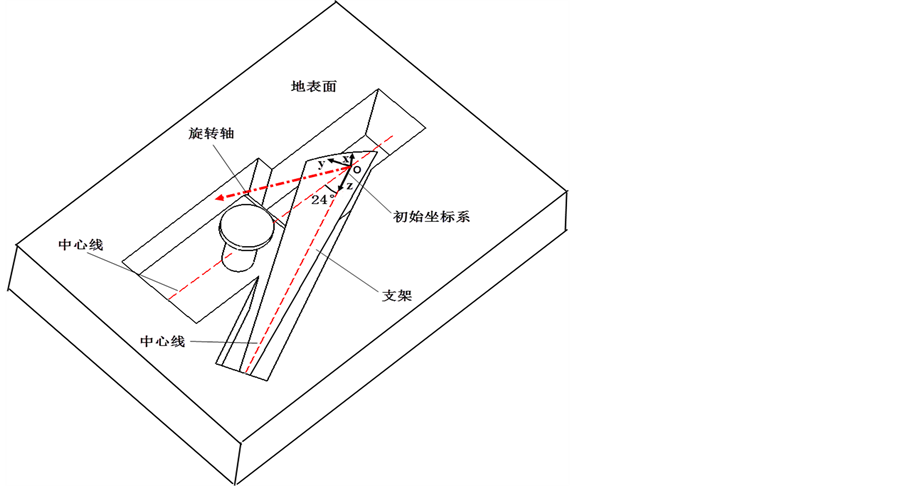(a) 支架放倒时的初始位姿及人字形地坑夹角 (b) 支架起竖时的位姿

Figure 2. The configuration when the pylon is laid down and erected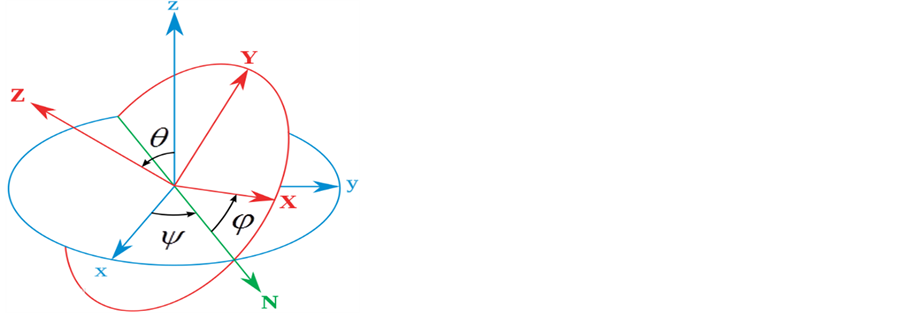Figure 3. The Euler angle formed by the initial and final coordinate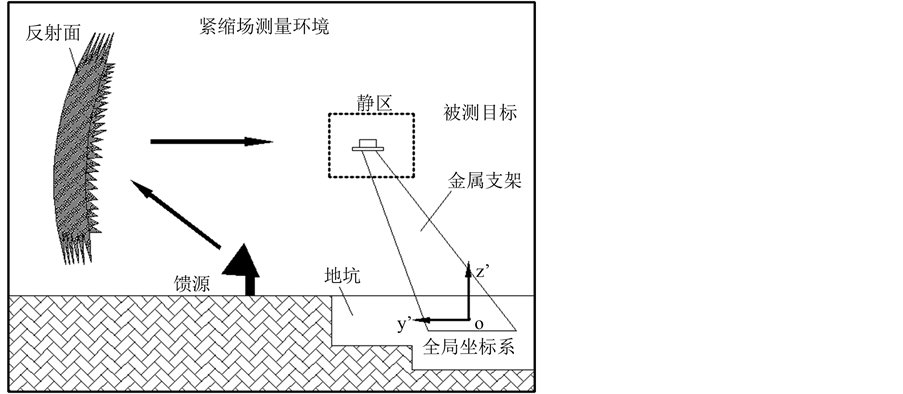Figure 4. The global coordinate system of compact field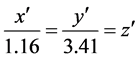4. 对支架进行力学仿真

4.1. 刚、强度分析

4.2. 动力学分析Table 1. Static analysis results and safety factor calculation of the pylon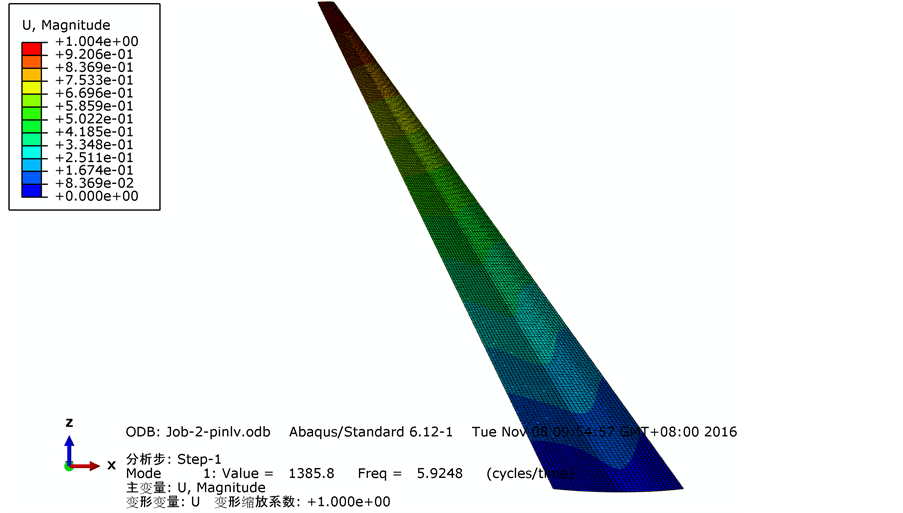(a) 一阶模态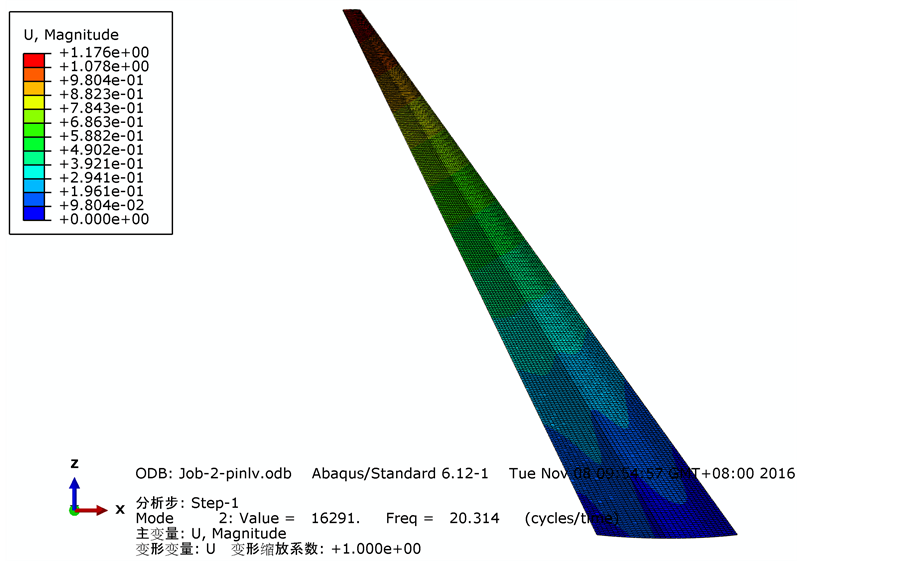(b) 二阶模态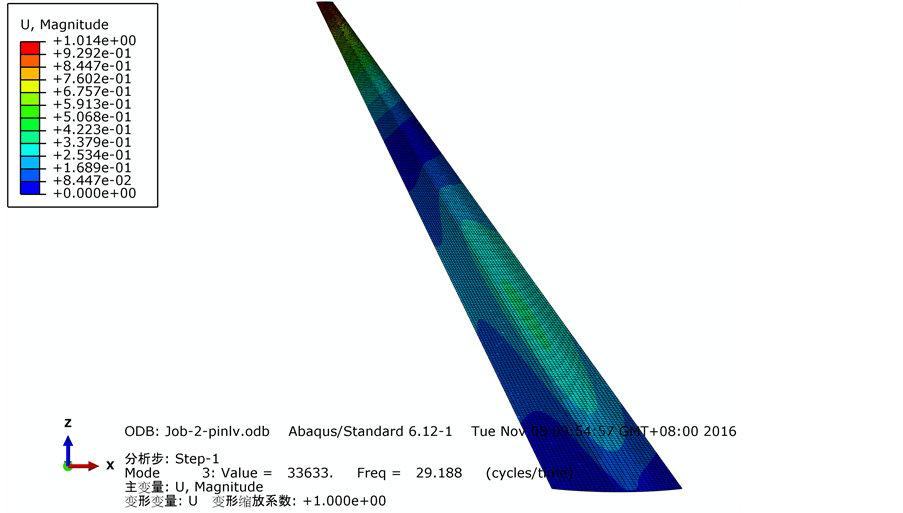(c) 三阶模态

Figure 5. Modal shapes of various orders

6. 结论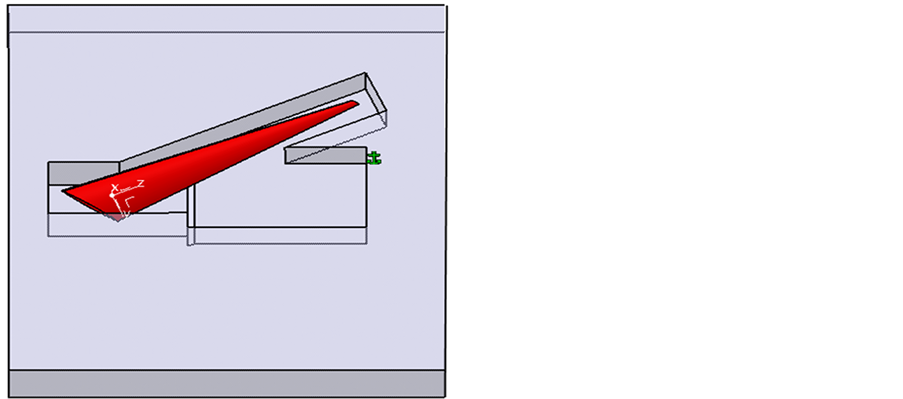Figure 6. The physical model established in CATIA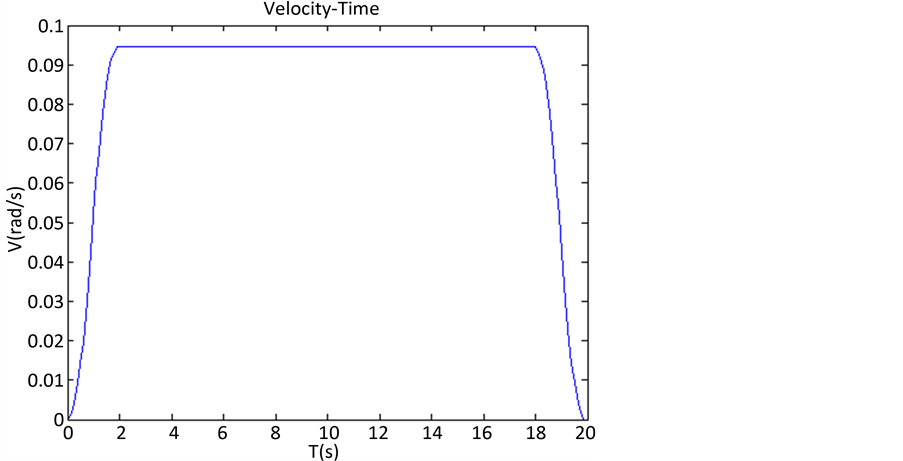Figure 7. The diagram of angular velocity of pylon with respect to time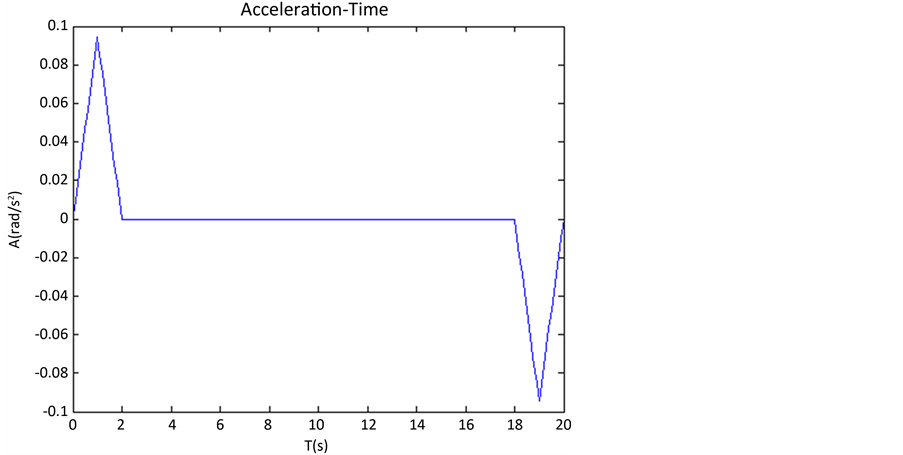Figure 8. The diagram of angular acceleration of pylon with respect to time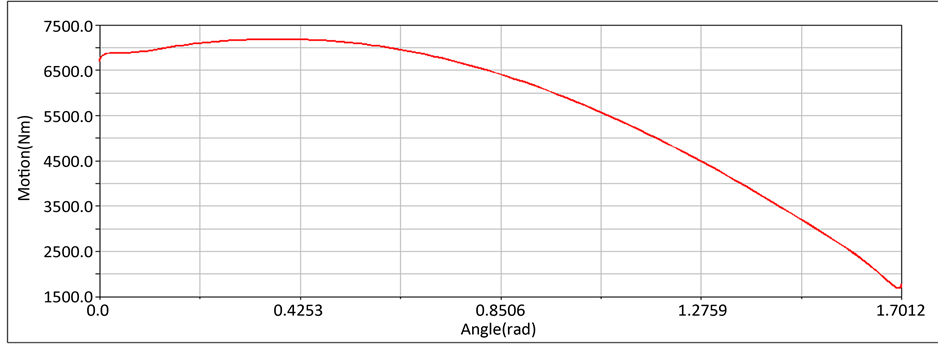Figure 9. The diagram of driving torque of pylon with respect to its angle

Kinematic Calculation and Simulation of a Low Scattering Metal Pylon[J]. 机械工程与技术, 2017, 06(01): 68-77. http://dx.doi.org/10.12677/MET.2017.61010

1. 1. 薛明华, 徐永斌. 遮掩式低回波金属支架的设计[J]. 微波学报, 1994(1): 51-54.

2. 2. 马永光. 关于RCS测量中若干问题的研究[D]: [博士学位论文]. 北京: 北京航空航天大学, 2009.

3. 3. Knott, E.F. (1993) Radar Cross Section Measurements. Proceedings of the IEEE, 27, 197.

4. 4. 焦洪杰, 陈五一, 张以都. 飞行器隐身测试用低散射塔架结构及其制造工艺[J]. 航空制造技术, 2009(4): 86-89.

5. 5. 焦洪杰. 紧缩场低散射塔架结构工程化设计研究[A]. Beihang University. 机械技术史及机械设计(6)——第六届中日机械技术史及机械设计国际学术会议论文集[C]. Beihang University, 2006: 6.

6. 6. 唐海正, 徐长龙, 徐得名, 等. 一种新型目标支架的设计和分析[J]. 微波学报, 2000, 16(4): 434-439.

7. 7. 唐海正, 徐长龙, 徐得名. 一种新型目标支架的设计和理论分析[J]. 上海大学学报(自然科学版). 2000(1): 11-14.

8. 8. 安大卫, 李志平, 陈五一. 低散射目标支撑金属支架的外形参数优化[J]. 电讯技术, 2015(3): 333-339.